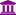# Centroid calculation with K-means using nominal measures

Member Posts: 2Contributor I
edited July 2019 in Help

Hello,

I am using the K-means algorithm in order to do a clustering process where all the attributes that I have are nominal. The clustering process seems to work well but when I try to obtain the prototype of each cluster using the "Extract cluster prototype" the result that I obtain is not clear to me. I think that the centroid of a set of samples with nominal data is usually calculated as the mode (most frequent value) of each attribute but the result obtained with the "Extract cluster prototype" is not the same as the mode. How is the cluster prototype calculated with nominal data?

Can anybody help me? Thank you very much in advance.

Best Regards.

Juan.

Tagged:

• Administrator, Moderator, Employee, RapidMiner Certified Analyst, RapidMiner Certified Expert, University Professor Posts: 3,363RM Data Scientist
Solution Accepted

Dear  juansanchez,

the point about K-Means is, that it always does it's thing. It's thing is to get the best centeroid. The idea behind the whole algorithm is to find the cluster with the smallest variance. Variance translates to euclidian distance and thus it optimizes to find the cluster with the small in-cluster variance (=smallest average euclidian distance). The result is always a centeroid, which might not be a real data point (or even a valid point for integers or strings). But this is how it goes.

If you do not use euclidian distance in k-Means the algorithm is neither garunteed to converge nor is it following it's key principals (=variance minimization).

I've confused the algorithm names a bit, sorry for this. The algorithm i would propose is k-Medoids.

~Martin

- Head of Data Science Services at RapidMiner -
Dortmund, Germany

• Administrator, Moderator, Employee, RapidMiner Certified Analyst, RapidMiner Certified Expert, University Professor Posts: 3,363RM Data Scientist

I would strongly encourage you not to use K-Means but K-Medians if you have a nominal distance measure. K-Means is usually only defined for Euclidian distance.

~Martin

- Head of Data Science Services at RapidMiner -
Dortmund, Germany
• Member Posts: 2Contributor I

Hello again and thanks for your response.

I do not find the K-medians process in my version of Rapidminer. In any case, with K-means, since I am working with categorical data (or equivalently nominal data), I use the "Nominal distance" that seems to calculate the distance as the number of elements that are different between the two vectors (i.e. Hamming distance). For example, if I have a vector v1={B,B,B} and a vector v2={A,B,A}, the distance is 2.

The thing is that I do not know how the centroid is calculated in the "extract prototype" process in Rapidminer with K-means  with categorical data and Hamming distance. For example if I have a cluster with the vectors:

v1={B,B,B}

v2={A,B,A}

v3={A,A,B}

I would say that the cluster prototype would be {A,B,B} and I determine this vector by calculating the mode (most frequent value) of the each element of the three vectors. The mode is usually considered for the calculation of the centroid with this kind of data. The result obtained with the "Extract cluster prototype" process in this case is {B,B,B}. I don't understand how this prototype is determined. In this case, the prototype corresponds to one of the vectors but in other cases that I have tested it doesn't.

Best regards.

Juan.

• Member, University Professor Posts: 1University Professor

hi

I'm not sure that the problem is not a bug with the coding/decoding of the nominal variables. I prepared an example which I think illustrates this.

I generated some data with 2 clear clusters

cluster 1 with 50 examples

-v1: if rand < 0.3, "b", else if rand < 0.3, "c", else "a" (polynomial) (mostly "a" but 30% probability of being "b", 30%*70% of being "c")

-v2: if rand < 0.3, "y", else "x" (binomial) (mostly "x" but 30% probability of being "y")

-v3: uniformly distributed between 5 and 15

cluster 2 with 51 examples

-v1: if rand < 0.3, "a", else if rand < 0.3, "c", else "b" (polynomial) (mostly "b" but 30% probability of being "a", 30%*70% of being "c")

-v2: if rand < 0.3, "x", else "y" (binomial) (mostly "y" but 30% probability of being "x")

-v3: uniformly distributed between 45 and 55

You can find an instance of the data in worksheet "dataset" of the attached file (the generation process is in worksheet "generation").

Worksheet "analysis" shows that the clusters are clear cut and the k-means (also the k-medoids) can find them easily.

Then, I created a mapping between nominal and integer values for each nominal variable: 1st value observed in the data is converted to 0, 2nd to 1, ... I used the mapping of each variable to convert it to an integer variable. This can be found in worksheet "dataset (analysis version)".

Then I compared the centroids of the clustering (see attached figure) with the expected values (computed directly from the data in the dataset with translated values - worksheet "analysis") and they match.

The operator Extract Cluster Prototypes (see attached figure) returns:

-the first cluster is correct

-the second is not, for the nominal variables (should be "b" and "y")

If I'm missing something, I appreciate if somebody can help.

best regards,

Carlos